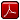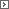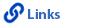Mathematics Grade 4Printable Version (pdf)Course Introduction

Core Standards of the Course

Strand: Mathematical PRACTICES (4.MP)
The Standards for Mathematical Practice in Fourth Grade describe mathematical habits of mind that teachers should seek to develop in their students. Students become mathematically proficient in engaging with mathematical content and concepts as they learn, experience, and apply these skills and attitudes (Standards 4.MP.1-8).Standard 4.MP.1
Make sense of problems and persevere in solving them. Explain the meaning of a problem, look for entry points to begin work on the problem, and plan and choose a solution pathway. When a solution pathway does not make sense, look for another pathway that does. Explain connections between various solution strategies and representations. Upon finding a solution, look back at the problem to determine whether the solution is reasonable and accurate, often checking answers to problems using a different method or approach.

Standard 4.MP.2
Reason abstractly and quantitatively. Make sense of quantities and their relationships in problem situations. Contextualize quantities and operations by using images or stories. Decontextualize a given situation and represent it symbolically. Interpret symbols as having meaning, not just as directions to carry out a procedure. Know and flexibly use different properties of operations, numbers, and geometric objects.

Standard 4.MP.3
Construct viable arguments and critique the reasoning of others. Use stated assumptions, definitions, and previously established results to construct arguments. Explain and justify the mathematical reasoning underlying a strategy, solution, or conjecture by using concrete referents such as objects, drawings, diagrams, and actions. Listen to or read the arguments of others, decide whether they make sense, ask useful questions to clarify or improve the arguments, and build on those arguments.

Standard 4.MP.4
Model with mathematics. Identify the mathematical elements of a situation and create a mathematical model that shows the relationships among them. Identify important quantities in a contextual situation, use mathematical models to show the relationships of those quantities, analyze the relationships, and draw conclusions. Models may be verbal, contextual, visual, symbolic, or physical.

Standard 4.MP.5
Use appropriate tools strategically. Consider the tools that are available when solving a mathematical problem, whether in a real-world or mathematical context. Choose tools that are relevant and useful to the problem at hand, such as drawings, diagrams, technologies, and physical objects and tools, as well as mathematical tools such as estimation or a particular strategy or algorithm.

Standard 4.MP.6
Attend to precision. Communicate precisely to others by crafting careful explanations that communicate mathematical reasoning by referring specifically to each important mathematical element, describing the relationships among them, and connecting their words clearly to representations. Calculate accurately and efficiently, and use clear and concise notation to record work.

Standard 4.MP.7
Look for and make use of structure. Recognize and apply the structures of mathematics such as patterns, place value, the properties of operations, or the flexibility of numbers. See complicated things as single objects or as being composed of several objects.

Standard 4.MP.8
Look for and express regularity in repeated reasoning. Notice repetitions in mathematics when solving multiple related problems. Use observations and reasoning to find shortcuts or generalizations. Evaluate the reasonableness of intermediate results.

Strand: OPERATIONS AND ALGEBRAIC THINKING (4.OA)
Use the four operations with whole numbers (addition, subtraction, multiplication, and division) to solve problems (Standards 4.OA.1-3). Gain familiarity with factors and multiples (Standard 4.OA.4). Generate and analyze numeric and shape patterns (Standard 4.OA.5).Standard 4.OA.1
Interpret a multiplication equation as a comparison (for example, interpret 35 = 5 x 7 as a statement that 35 is 5 times as many as 7 and 7 times as many as 5). Represent verbal statements of multiplicative comparisons as multiplication equations.Standard 4.OA.2
Multiply or divide to solve word problems involving multiplicative comparison, for example, by using drawings and equations with a symbol for the unknown number to represent the problem, distinguishing multiplicative comparison from additive comparison.Standard 4.OA.3
Solve multi-step word problems posed with whole numbers and having whole-number answers using the four operations, including problems in which remainders must be interpreted.1. Represent these problems using equations with a letter standing for the unknown quantity.
2. Assess the reasonableness of answers using mental computation and estimation strategies, including rounding.

Standard 4.OA.4
Find all factor pairs for a whole number in the range 1-100. Recognize that a whole number is a multiple of each of its factors. Determine whether a given whole number in the range 1-100 is a multiple of a given one-digit number. Determine whether a given whole number in the range 1-100 is prime or composite.Standard 4.OA.5
Generate a number or shape pattern that follows a given rule. Identify apparent features of the pattern that were not explicit in the rule itself. For example, given the rule "add 3" and the starting number 1, generate terms in the resulting sequence and observe that the terms appear to alternate between odd and even numbers. Explain informally why the numbers will continue to alternate in this way.Strand: NUMBER AND OPERATIONS IN BASE TEN (4.NBT)
Generalize place value understanding for multi-digit whole numbers by analyzing patterns, writing whole numbers in a variety of ways, making comparisons, and rounding (Standards 4.NBT.1-3). Use place value understanding and properties of operations to perform multidigit addition, subtraction, multiplication, and division using a one-digit divisor (Standards 4.NBT.4-6). Expectations in this strand are limited to whole numbers less than or equal to 1,000,000.Standard 4.NBT.1
Recognize that in a multi-digit whole number, a digit in one place represents ten times what it represents in the place to its right. For example, recognize that 700 � 70 = 10 by applying concepts of place value and division.Standard 4.NBT.2
Read and write multi-digit whole numbers using base-ten numerals, number names, and expanded form. Compare two multi-digit numbers based on meanings of the digits in each place, using >, =, and < symbols to record the results of comparisons.Standard 4.NBT.3
Use place value understanding to round multi-digit whole numbers to any place.Standard 4.NBT.4
Fluently add and subtract multi-digit whole numbers using the standard algorithm.Standard 4.NBT.5
Multiply a whole number of up to four digits by a one-digit whole number, and multiply two two-digit numbers, using strategies based on place value and the properties of operations. Illustrate and explain the calculation by using equations, rectangular arrays, and/or area models.Standard 4.NBT.6
Find whole-number quotients and remainders with up to four-digit dividends and one-digit divisors, using strategies based on place value, the properties of operations, and/or the relationship between multiplication and division. Illustrate and explain the calculation by using equations, rectangular arrays, and/or area models.Strand: NUMBER AND OPERATIONS - FRACTIONS (4.NF)
Extend understanding of equivalence and ordering of fractions (Standards 4.NF.1-2). Build fractions from unit fractions by applying and extending previous understandings of operations on whole numbers (Standards 4.NF.3-4). Understand decimal notation to the hundredths and compare decimal fractions with denominators of 10 and 100 (Standards 4.NF.5- 7). Denominators for fourth grade are limited to 2, 3, 4, 5, 6, 8, 10, 12, and 100.Standard 4.NF.1
Explain why a fraction a/b is equivalent to a fraction (n x a)/(n x b) by using visual fraction models, with attention to how the number and size of the parts differ even though the two fractions themselves are the same size. Use this principle to recognize and generate equivalent fractions.Standard 4.NF.2
Compare two fractions with different numerators and different denominators, for example, by creating common denominators or numerators, or by comparing to a benchmark fraction such as 1/2. Recognize that comparisons are valid only when the two fractions refer to the same whole. Record the results of comparisons with symbols >, =, or <, and justify the conclusions, for example, by using a visual fraction model.Standard 4.NF.3
Understand a fraction a/b with a >1 as a sum of fractions 1/b. In other words, any fraction is a sum of unit fractions.1. Understand addition and subtraction of fractions as joining and separating parts referring to the same whole.
2. Decompose a fraction into a sum of fractions with the same denominator in more than one way, recording each decomposition by an equation. Justify decompositions, for example, by using a visual fraction model. For example, 3/8 = 1/8 + 1/8 + 1/8; 3/8 = 1/8 + 2/8; 2 1/8 = 1 + 1 + 1/8; 2 1/8 = 8/8 + 8/8 + 1/8.
3. Add and subtract mixed numbers with like denominators, for example, by replacing each mixed number with an equivalent fraction, and/or by using properties of operations and the relationship between addition and subtraction. For example, 3 1/4 + 2 1/4 = 13/4 + 9/4 = 22/4; 3 1/4 + 2 1/4 = (3+ 2) + (1/4 + 1/4) = 5 + 2/4 = 5 2/4, which is equivalent to 22/4.
4. Solve word problems involving addition and subtraction of fractions referring to the same whole and having like denominators, for example, by using visual fraction models and equations to represent the problem.

Standard 4.NF.4
Apply and extend previous understandings of multiplication to multiply a fraction by a whole number.1. Understand a fraction a/b as a multiple of 1/b. For example, use a visual fraction model to represent 5/4 as the product 5 x (1/4), recording the conclusion by the equation 5/4 = 5 x (1/4).
2. Understand a multiple of a/b as a multiple of 1/b, and use this understanding to multiply a fraction by a whole number. For example, use a visual fraction model to express 3 x (2/5) as 6 x (1/5), recognizing this product as 6/5. (In general, n x (a/b) = (n x a)/b).
3. Solve word problems involving multiplication of a fraction by a whole number (for example, by using visual fraction models and equations to represent the problem). For example, if each person at a party will eat 3/8 of a pound of roast beef, and there will be five people at the party, how many pounds of roast beef will be needed? Between what two whole numbers does your answer lie?

Standard 4.NF.5
Express a fraction with denominator 10 as an equivalent fraction with denominator 100, and use this technique to add two fractions with respective denominators 10 and 100. For example, express 3/10 as 30/100, and add 3/10 + 4/100 = 34/100.Standard 4.NF.6
Use decimal notation for fractions with denominators 10 or 100. For example, rewrite 0.62 as 62/100, describe a length as 0.62 meters; locate 0.62 on a number line diagram.Standard 4.NF.7
Compare two decimals to hundredths by reasoning about their size. Recognize that comparisons are valid only when the two decimals refer to the same whole. Record the results of comparisons with the symbols >, =, or <, and justify the conclusions, for example, by using a visual model.Strand: MEASUREMENT AND DATA (4.MD)
Solve problems involving measurement and conversion of measurements from a larger unit to a smaller unit (Standards 4.MD.1-2). Apply knowledge of area and perimeter to solve realworld and mathematical problems (Standard 4.MD.3). Represent and interpret data through the use of a line plot (Standard 4.MD.4). Understand various concepts of angles and angle measurement (Standard 4.MD.5-7).Standard 4.MD.1
Know relative sizes of measurement units within each system of units (standard and metric), including kilometers, meters, and centimeters; liters and milliliters; kilograms and grams; pounds and ounces; hours, minutes, and seconds. Within a single system of measurement, express measurements in a larger unit in terms of a smaller unit. Record measurement equivalents in a two-column table. For example, know that one foot is 12 times as long as one inch. Express the length of a four-foot snake as 48 inches. Know that one meter is 100 times as long as one centimeter. Generate a conversion table for feet and inches listing the number pairs (1, 12), (2, 24), (3, 36)...Standard 4.MD.2
Use the four operations to solve word problems involving distances, intervals of time, liquid volumes, masses of objects, and money.1. Include problems involving simple fractions or decimals, and problems that require expressing measurements given in a larger unit in terms of a smaller unit.
2. Represent measurement quantities using diagrams such as number line diagrams that feature a measurement scale.

Standard 4.MD.3
Apply the area and perimeter formulas for rectangles in real-world and mathematical problems. For example, find the width of a rectangular room given the area of the flooring and the length, by viewing the area formula as a multiplication equation with an unknown factor.Standard 4.MD.4
Make a line plot to display a data set of measurements in fractions of a unit (halves, quarters, and eighths). Solve problems involving addition and subtraction with like denominators of fractions by using information presented in line plots. For example, use a line plot to find and interpret the difference in length between the longest and shortest pencils in a classroom.Standard 4.MD.5
Recognize angles as geometric figures that are formed wherever two rays share a common endpoint, and understand concepts of angle measurement.1. Understand that an angle is measured with reference to a circle with its center at the common endpoint of the rays, by considering the fraction of the circular arc between the points where the two rays intersect the circle. An angle that turns through 1/360 of a circle is called a "one-degree angle," and can be used to measure other angles.
2. Understand that an angle that turns through n one-degree angles is said to have an angle measure of n degrees.

Standard 4.MD.6
Measure angles in whole-number degrees using a protractor. Sketch angles of specified measure.Standard 4.MD.71. Understand that when an angle is decomposed into non-overlapping parts, the angle measure of the whole is the sum of the angle measures of the parts.
2. Solve addition and subtraction problems to find unknown angles on a diagram in real-world and mathematical problems, for example by using an equation with a symbol for the unknown angle measure.

Strand: GEOMETRY (4.G.)
Draw and identify lines and angles, as well as classify shapes by properties of their lines and angles (Standards 4.G.1-3)Standard 4.G.1
Draw points, lines, line segments, rays, angles (right, acute, and obtuse), and perpendicular and parallel lines. Identify these in two-dimensional figures.Standard 4.G.2
Classify two-dimensional figures based on the presence or absence of parallel or perpendicular lines, or the presence or absence of angles of a specified size. Recognize right triangles as a category, and identify right triangles.Standard 4.G.3
Recognize a line of symmetry for a two-dimensional figure as a line across the figure such that the figure can be folded along the line into matching parts. Identify line-symmetric figures and draw lines of symmetry.http://www.uen.org - in partnership with Utah State Board of Education (USBE) and Utah System of Higher Education (USHE).  Send questions or comments to USBE Specialists - Trish  French or Molly  Basham and see the Mathematics - Elementary website. For general questions about Utah's Core Standards contact the Director - Jennifer  Throndsen.

These materials have been produced by and for the teachers of the State of Utah. Copies of these materials may be freely reproduced for teacher and classroom use. When distributing these materials, credit should be given to Utah State Board of Education. These materials may not be published, in whole or part, or in any other format, without the written permission of the Utah State Board of Education, 250 East 500 South, PO Box 144200, Salt Lake City, Utah 84114-4200.